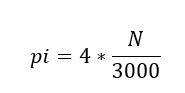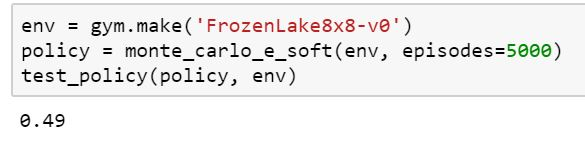# 强化学习：使用OpenAI Gym Toolkit进行蒙特卡洛学习简介

2年前 阅读 364 点赞 0## 目录

1. Model-Based 和 Model-Free Learning
2. 蒙特卡罗方法 - 示例
3. 蒙特卡洛强化学习
• Monte Carlo Prediction
• Monte Carlo Control

4.使用Python的OpenAI Gym工具包

## Model-Based 和 Model-Free Learning## 蒙特卡罗方法 - 示例## 蒙特卡洛策略评估

S1，A1，R2，... .Sk~pi• i - 片段指数
• s - 状态指数

First Visit Monte Carlo 在一个片段中 s 第一次被访问的平均回报率。

1. 初始化策略，状态值函数
2. 首先根据当前策略生成片段
3. 跟踪通过该片段遇到的状态
4. 在2.a中选择一个状态
5. 将首次出现此状态后收到的回报添加到列表中
6. 所有回报的平均值
7. 将状态值设置为计算的平均值
8. 重复步骤3
9. 重复2-4直到满意为止

Every visit Monte Carlo 在某一片段中中每次访问 s 的平均回报。A + 3 => A表示从状态A到状态A的转换，奖励+3。让我们使用两种方法找出值函数：### 增量平均值V（S t）←V（S t）+α（G t - V（S t））

## Monte Carlo Control## Python中的蒙特卡罗实现

### Frozen Lake Environment（S：起点，安全），（F：冻结表面，安全），（H：洞，落入你的厄运），（G：目标）

import gym
import numpy as np
import operator
from IPython.display import clear_output
from time import sleep
import random
import itertools
import tqdm

tqdm.monitor_interval = 0


def create_random_policy(env):
policy = {}
for key in range(0, env.observation_space.n):
current_end = 0
p = {}
for action in range(0, env.action_space.n):
p[action] = 1 / env.action_space.n
policy[key] = p
return policy


def create_state_action_dictionary(env, policy):
Q = {}
for key in policy.keys():
Q[key] = {a: 0.0 for a in range(0, env.action_space.n)}
return Q


def run_game(env, policy, display=True):
env.reset()
episode = []
finished = False

while not finished:
s = env.env.s
if display:
clear_output(True)
env.render()
sleep(1)

timestep = []
timestep.append(s)
n = random.uniform(0, sum(policy[s].values()))
top_range = 0
for prob in policy[s].items():
top_range += prob
if n < top_range:
action = prob
break
state, reward, finished, info = env.step(action)
timestep.append(action)
timestep.append(reward)

episode.append(timestep)

if display:
clear_output(True)
env.render()
sleep(1)
return episode


def test_policy(policy, env):
wins = 0
r = 100
for i in range(r):
w = run_game(env, policy, display=False)[-1][-1]
if w == 1:
wins += 1
return wins / r


def monte_carlo_e_soft(env, episodes=100, policy=None, epsilon=0.01):
if not policy:
policy = create_random_policy(env)  # Create an empty dictionary to store state action values
Q = create_state_action_dictionary(env, policy) # Empty dictionary for storing rewards for each state-action pair
returns = {} # 3.

for _ in range(episodes): # Looping through episodes
G = 0 # Store cumulative reward in G (initialized at 0)
episode = run_game(env=env, policy=policy, display=False) # Store state, action and value respectively

# for loop through reversed indices of episode array.
# The logic behind it being reversed is that the eventual reward would be at the end.
# So we have to go back from the last timestep to the first one propagating result from the future.

for i in reversed(range(0, len(episode))):
s_t, a_t, r_t = episode[i]
state_action = (s_t, a_t)
G += r_t # Increment total reward by reward on current timestep

if not state_action in [(x, x) for x in episode[0:i]]: #
if returns.get(state_action):
returns[state_action].append(G)
else:
returns[state_action] = [G]

Q[s_t][a_t] = sum(returns[state_action]) / len(returns[state_action]) # Average reward across episodes

Q_list = list(map(lambda x: x, Q[s_t].items())) # Finding the action with maximum value
indices = [i for i, x in enumerate(Q_list) if x == max(Q_list)]
max_Q = random.choice(indices)

A_star = max_Q # 14.

for a in policy[s_t].items(): # Update action probability for s_t in policy
if a == A_star:
policy[s_t][a] = 1 - epsilon + (epsilon / abs(sum(policy[s_t].values())))
else:
policy[s_t][a] = (epsilon / abs(sum(policy[s_t].values())))

return policy